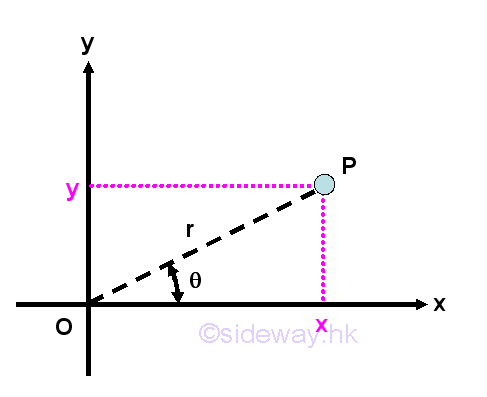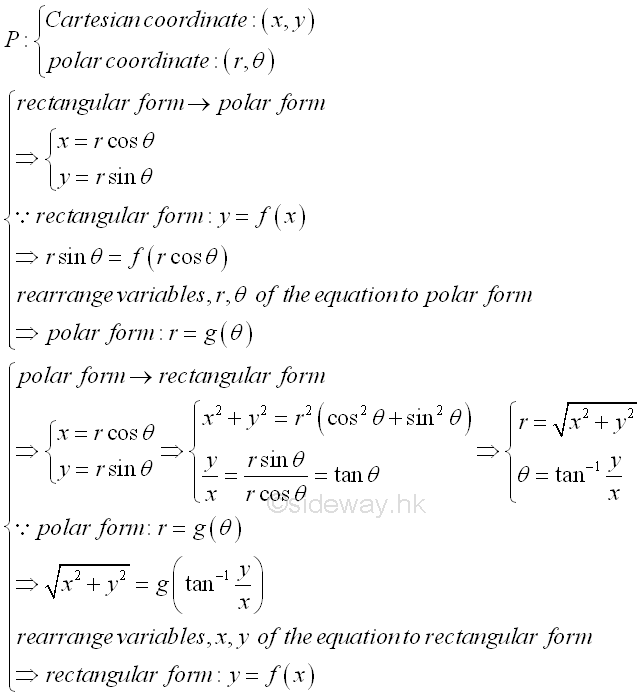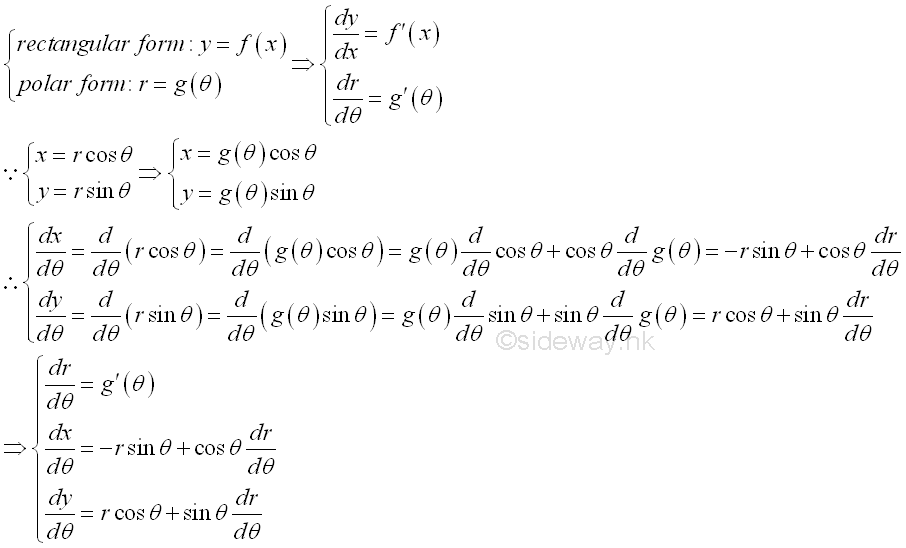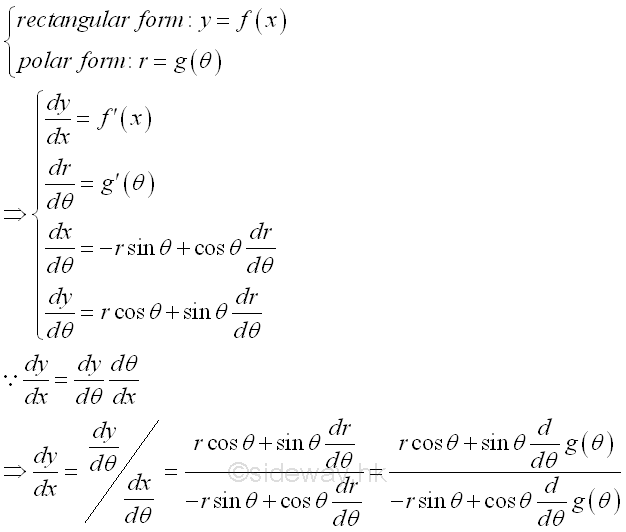output.to from Sideway
Draft for Information Only

# Content

```Polar Equation  Derivative of Polar Equation  Derivative of Cartesian Equation from Polar form```

# Polar Equation

Besides the parametric form of a curve representation that using a parameter to locate the coordinates (x,y) of point p on the Cartesian plane as a function of one variable, t called parameter. A polar form can also be used to describe a curve on the Cartesian plane using the polar coordinates (r,θ) with the positive x-axis as the fixed initial line. line joining the origin and point P is the radius, r and angle formed by line OP and the fixed initial line OX  is the angle θ. Similar to rectangular coordinate system that y is a function of x, radius r is also a function of angle θ in polar coordinate system,and the polar form of a curve is r=f(θ). ImplySince P has both the polar coordinates (r,θ) with respect to the pole O and the initial line OX and has the Cartesian coordinates (x,y) with respect to the axes of reference OX and OY. the polar equation of the curve with the form  r=f(θ) can be obtained by substituting x=r cos θ , and y=r sin θ into the Cartesian equation of the curve after equating the values of Cartesian coordinates and Polar coordinates. Similarly, the Cartesian equation can also be obtained by substituting r=(x²+y²) and θ=tan⁻¹(y/x) into the Polar equation of the curve after equating the two equation x=r cos θ , and y=r sin θ. Imply## Derivative of Polar Equation

Unlike parametric equation, Leibniz’s notation is usually adopted  to denote the derivative of polar equation as in the rectangular equation. The main difference is polar equation is differentiating the polar equation with respect to θ while rectangular equation is differentiating the rectangular equation with respect to x. Imply## Derivative of Cartesian Equation from Polar form

Similar to parametric equation, the derivative of Cartesian equation from polar form can also be obtained from the related polar equations by making use of the rule of function of a function. ImplyID: 130700031 Last Updated: 7/17/2013 Revision: 0 Ref:References

1. S. James, 1999, Calculus
2. B. Joseph, 1978, University Mathematics: A Textbook for Students of Science &amp; EngineeringHome 5

Management

HBR 3

Information

Recreation

Culture

Chinese 1097

English 339

Reference 79Computer

Hardware 224Software

Application 213

Latex 52Manim 204

KB 1

Numeric 19

Programming

Web 289

Unicode 504

HTML 66

CSS 65

SVG 46

ASP.NET 270

OS 429

Python 72

Knowledge

Mathematics

Algebra 84

Geometry 33Calculus 67

Engineering

Mechanical

Rigid Bodies

Statics 92

Dynamics 37

Control

Natural Sciences

Electric 27